Question

# Given these reactions, X(s)+1/2O2(g) >>> XO(s) Delta H -853.9 kj XCO3(s) >>> XO(s)+CO2(g) Delta H +145.7...

Given these reactions, X(s)+1/2O2(g) >>> XO(s) Delta H -853.9 kj

XCO3(s) >>> XO(s)+CO2(g) Delta H +145.7 kj

what is DeltaHrxn for this reaction? X(s)+1/2O2(g)+CO2(g) >>> XCO3(s)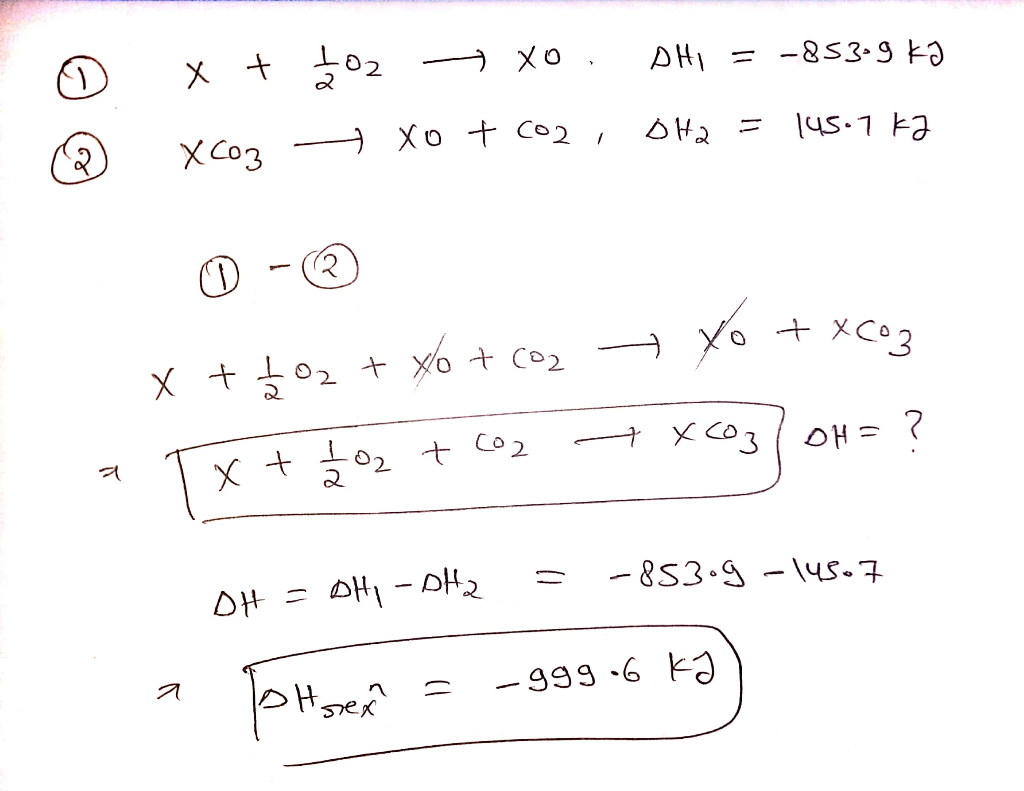#### Earn Coins

Coins can be redeemed for fabulous gifts.

Similar Homework Help Questions
• ### Given the reactions, - XO(S) AH = -571.5 kJ XCO3(s) — XO(s) + CO2(g) AH =...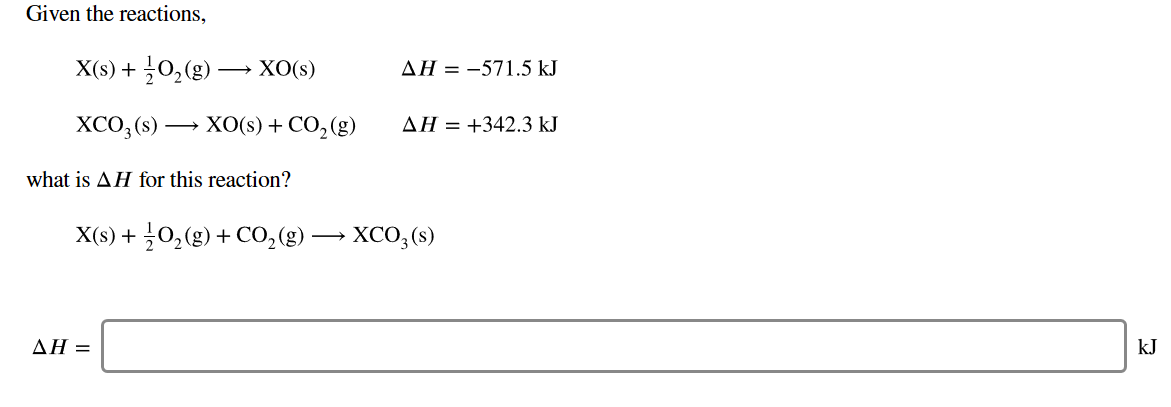Given the reactions, - XO(S) AH = -571.5 kJ XCO3(s) — XO(s) + CO2(g) AH = +342.3 kJ what is AH for this reaction? X(s) + 2O2(g) + CO2(g) → XCO3(s) AH =

• ### Given the reactions, → XO(s) AH = -593.1 kJ XCO3(s) — XO(s) + CO2(8) AH =...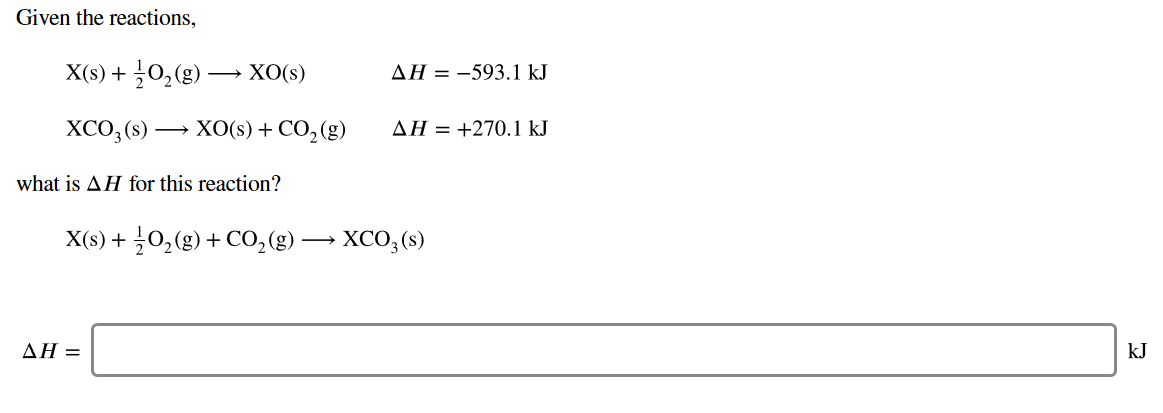Given the reactions, → XO(s) AH = -593.1 kJ XCO3(s) — XO(s) + CO2(8) AH = +270.1 kJ what is AH for this reaction? X(s) + 2O2(g) + CO2(g) → XCO3(s) AH=

• ### Given the reactions, = -906.9 kJ X(s) + O2(g) + XO(s) XCO3(s) XO(s) + CO2(g) H...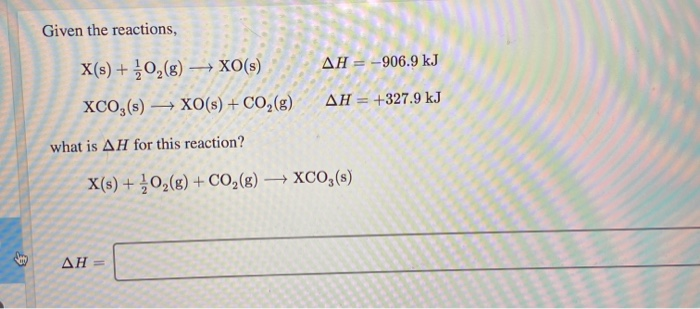Given the reactions, = -906.9 kJ X(s) + O2(g) + XO(s) XCO3(s) XO(s) + CO2(g) H = +327 what is AH for this reaction? X(s) + 302(8) + CO2(g) → XCO3(s) Ah=

• ### Given the reactions, X(s)+12O2(g)⟶XO(s)Δ?=−983.5 kJX(s)+12O2(g)⟶XO(s)ΔH=−983.5 kJ XCO3(s)⟶XO(s)+CO2(g)Δ?=+253.5 kJXCO3(s)⟶XO(s)+CO2(g)ΔH=+253.5 kJ what is Δ? for this reaction? X(s)+12O2(g)+CO2(g)⟶XCO3(s)

Given the reactions, X(s)+12O2(g)⟶XO(s)Δ?=−983.5 kJX(s)+12O2(g)⟶XO(s)ΔH=−983.5 kJ XCO3(s)⟶XO(s)+CO2(g)Δ?=+253.5 kJXCO3(s)⟶XO(s)+CO2(g)ΔH=+253.5 kJ what is Δ? for this reaction? X(s)+12O2(g)+CO2(g)⟶XCO3(s)

• ### Given these reactions 1 X(s) ХO(S) AH = -908.1 kJ/mol XCO3(s) XO(s)CO2(g) AH = +422.9 kJ/mol...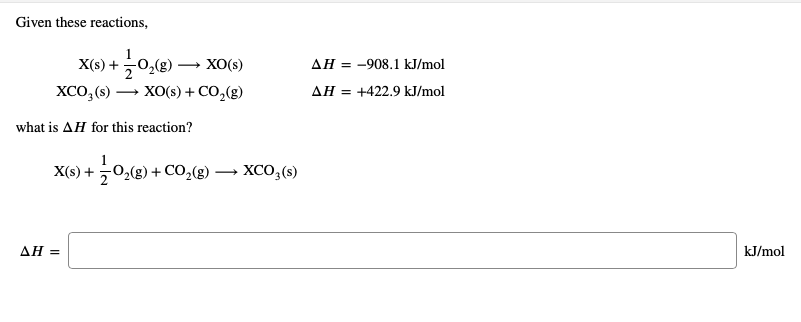Given these reactions 1 X(s) ХO(S) AH = -908.1 kJ/mol XCO3(s) XO(s)CO2(g) AH = +422.9 kJ/mol what is AH for this reaction? ХCО,(6) X(s) 2(g)CO2(g) АН — kJ/mol

• ### what is Δ?ΔH for this reaction? Given the reactions X(s)02(g) ΔΗ-924.3 k XO(s) XCO3 (s)XO(s) +...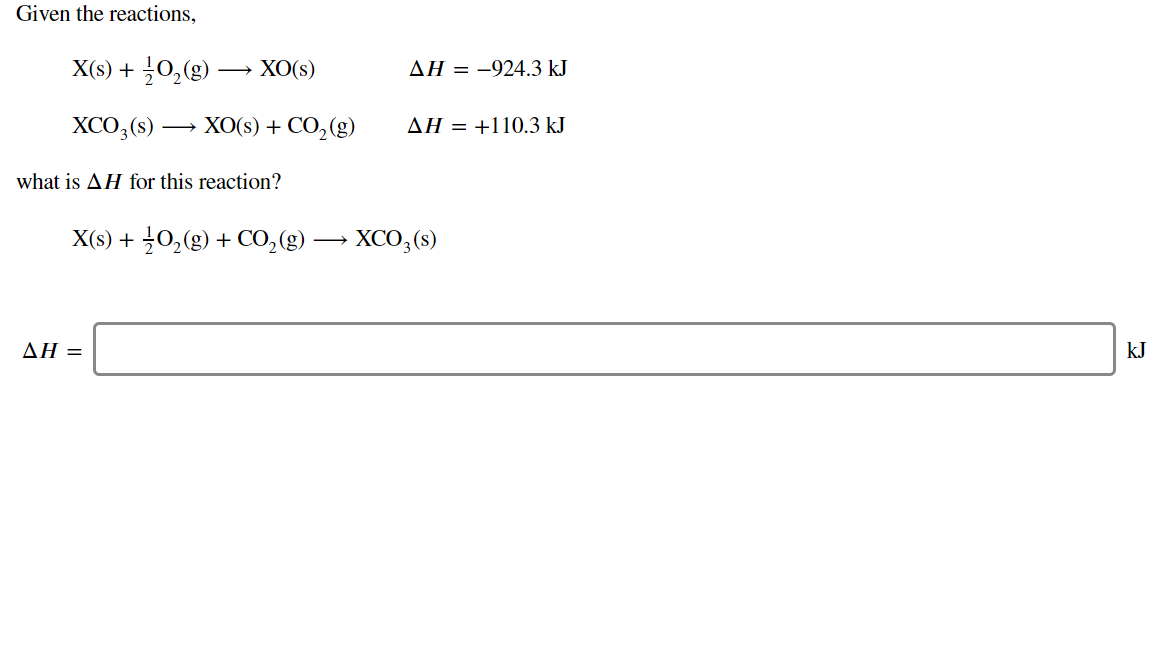what is Δ?ΔH for this reaction? Given the reactions X(s)02(g) ΔΗ-924.3 k XO(s) XCO3 (s)XO(s) + CO2 (g) ΔΗ+110.3 kJ what is AH for this reaction? X(s) 02(g)CO2(g) XCO,(s) ΔΗ= kJ

• ### Given the reactions, X(s) + O2(g) — XO(s) AH = -664.9 kJ XCO,() - XO(s) +...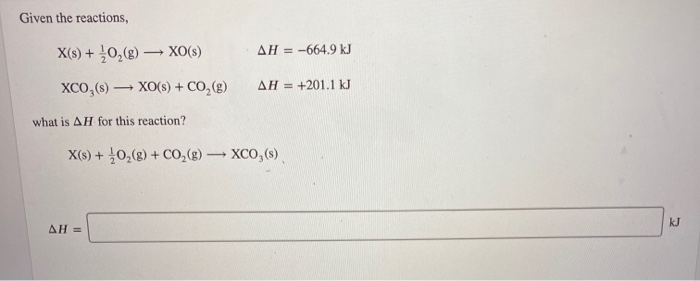Given the reactions, X(s) + O2(g) — XO(s) AH = -664.9 kJ XCO,() - XO(s) + CO2(g) AH = +201.1 kJ what is AH for this reaction? X(s) + 0,(8) + CO2(g) → XCO,(s) KJ AH =

• ### Given the reactions, X(s) + 0,(8) XO(s) XCO,(s) XO(s) + CO2(g) AH = -561.9 kJ AH...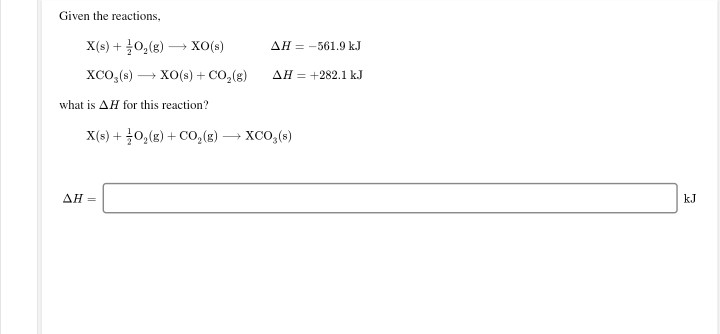Given the reactions, X(s) + 0,(8) XO(s) XCO,(s) XO(s) + CO2(g) AH = -561.9 kJ AH = +282.1 kJ what is AH for this reaction? X(s) + 0,(g) + CO,(g) XCO (5) AH=

• ### stion 21 of 25 > Given the reactions, X(s) + O2(g) → XO(s) XCO,(s) + XO(s)...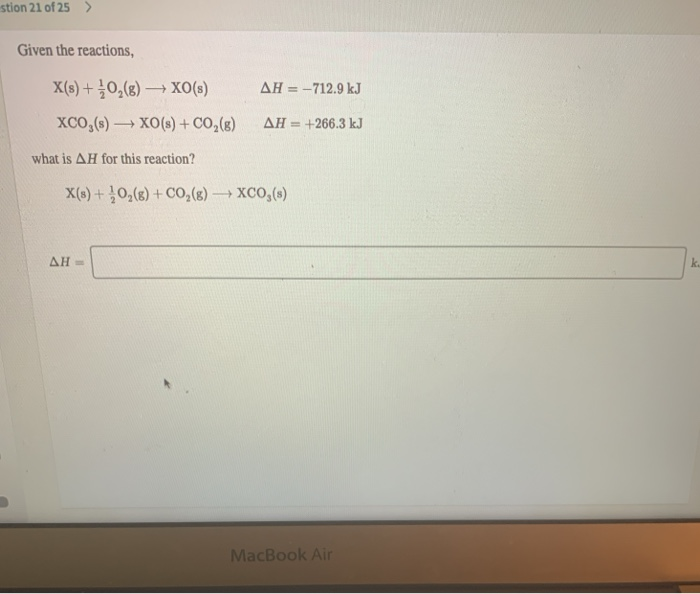stion 21 of 25 > Given the reactions, X(s) + O2(g) → XO(s) XCO,(s) + XO(s) + CO2(8) AH = -712.9 kJ AH = +266.3 kJ what is AH for this reaction? X(s) + O2(8) + CO2(g) → XCO,(s) AH- MacBook Air

• ### Given these reactions, X(s) + +0,8) — XO(S) XCO,(s) — XO(s) + CO2(9 what is AH...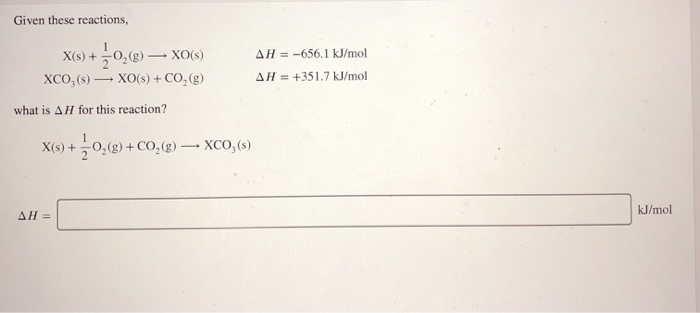Given these reactions, X(s) + +0,8) — XO(S) XCO,(s) — XO(s) + CO2(9 what is AH for this reaction? AH = -656.1 kJ/mol AH = +351.7 kJ/mol X(s) +0,() + CO2(g) — XCO,() AHL kJ/mol